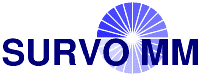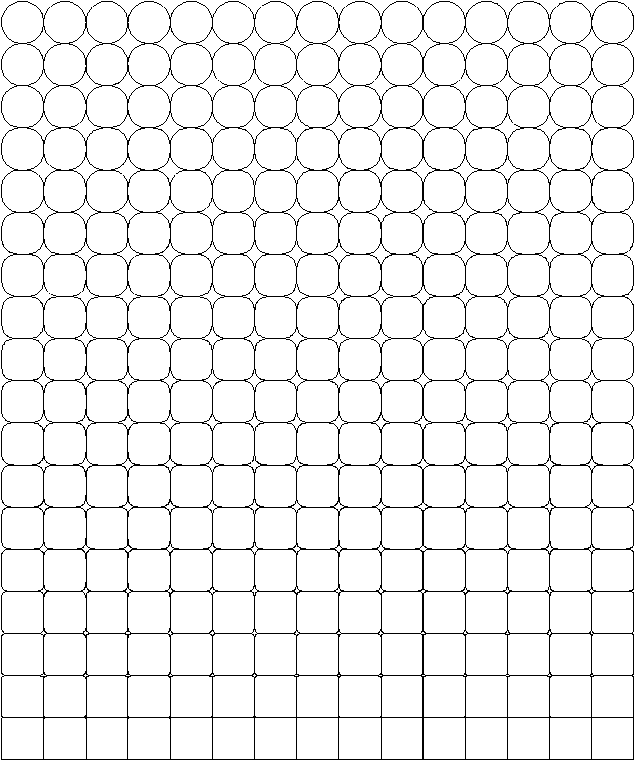# Environment for creative processing of text and numerical data## From circle to square

S.Mustonen, Survo Users' Guide (1992), p. 333### Edit field:

```   1 *
2 *     `"From circle to square"`
3 *
4 *`PLOT X(T)=M+1-P+R*CT*ABS(CT)^A,`
5 *     `Y(T)=Q+R*ST*ABS(ST)^A`
7 *T=[line_width(0.48)],0.001,2*pi+0.001,pi/30 pi=3.14159
8 *FRAME=0 R=0.5 A=-1+(P+M*(Q-1))/(M*N)
9 *M=15 N=18 P=1,M,1 Q=1,N,1
10 *XSCALE=0.5,M+0.5 YSCALE=0.5,N+0.5
11 *XDIV=0,1,0 YDIV=0,1,0 SIZE=1164,1164*N/M
12 *

```